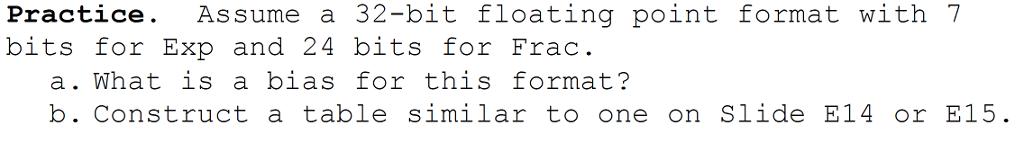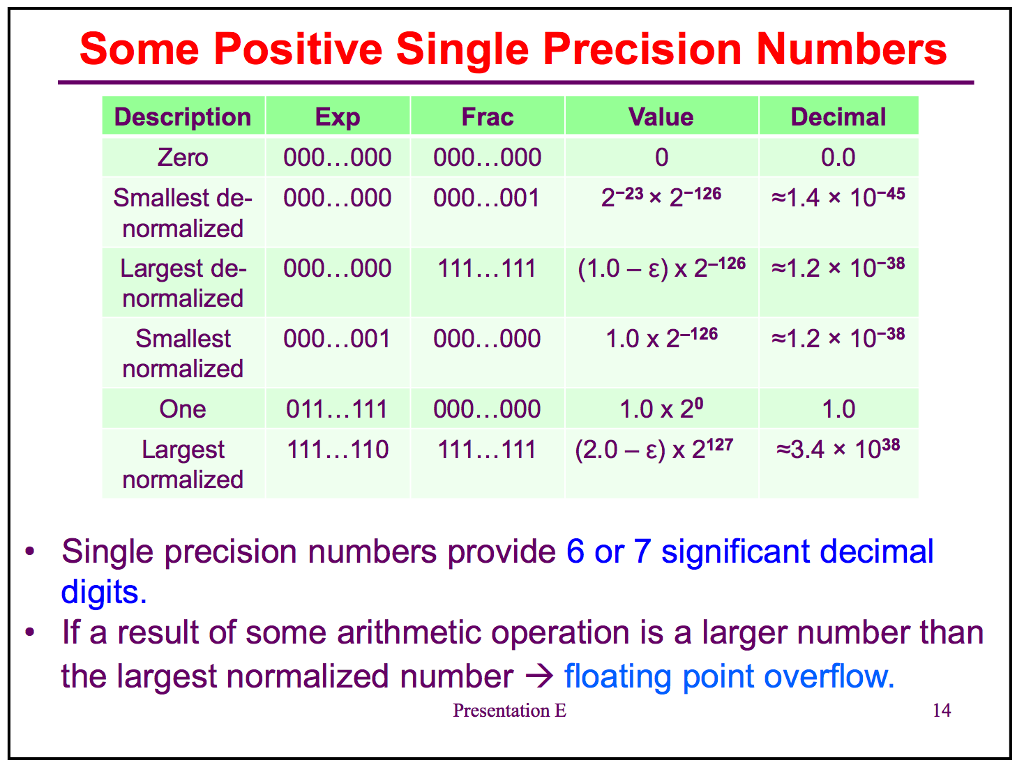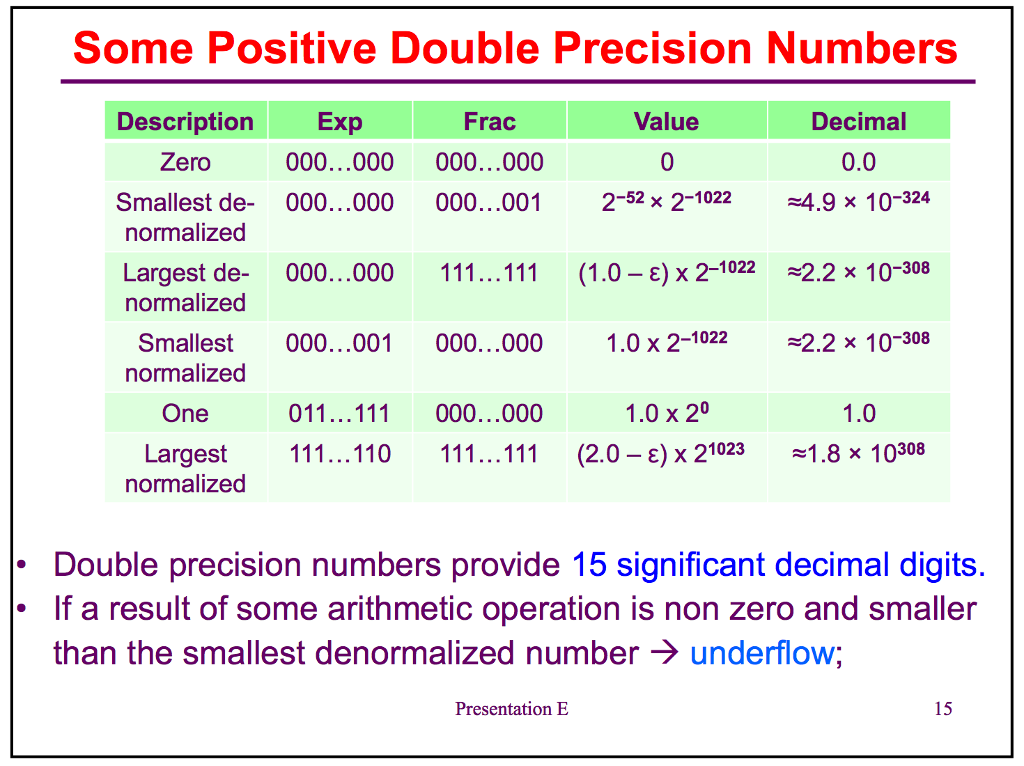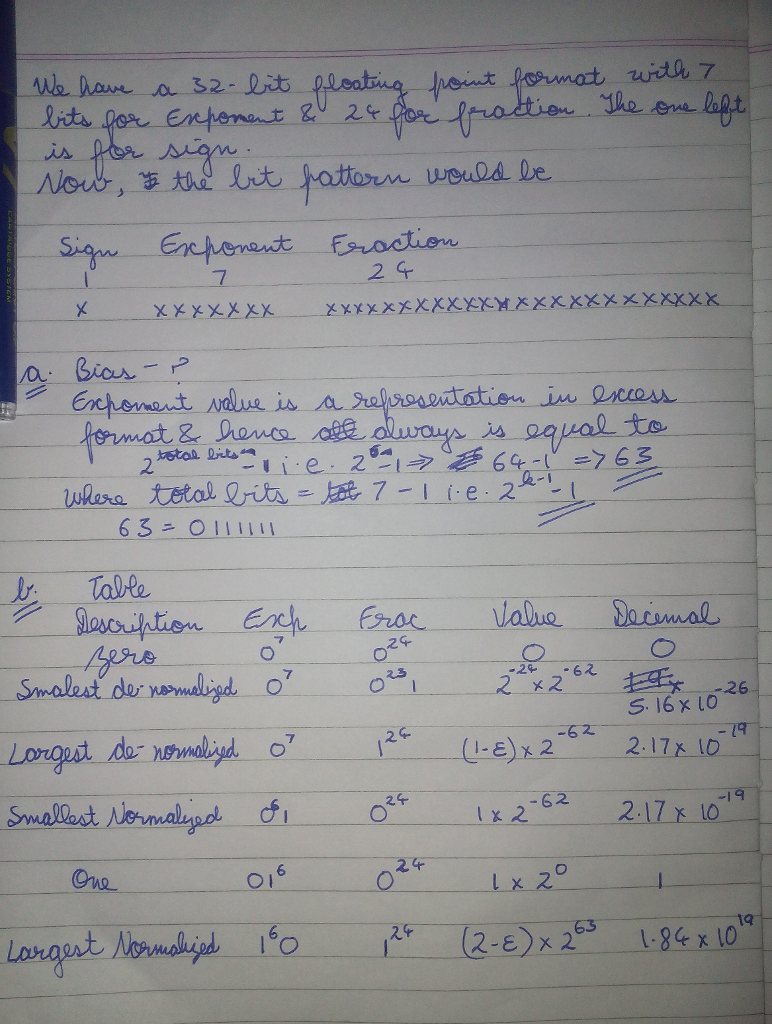# Answered! Assume a 32-bit floating point format with 7 bits for Exp and 24 bits for Frac. a. What is a bias for this format? b…Don't use plagiarized sources. Get Your Custom Essay on
Answered! Assume a 32-bit floating point format with 7 bits for Exp and 24 bits for Frac. a. What is a bias for this format? b…
GET AN ESSAY WRITTEN FOR YOU FROM AS LOW AS \$13/PAGE

Assume a 32-bit floating point format with 7 bits for Exp and 24 bits for Frac. a. What is a bias for this format? b. Construct a table similar to one on Slide E14 or E15. Single precision numbers provide 6 or 7 significant decimal digits. If a result of some arithmetic operation is a larger number than the largest normalized number rightarrow floating point overflow. Double precision numbers provide 15 significant decimal digits. If a result of some arithmetic operation is non zero and smaller than the smallest deformalized number rightarrow underflow;

It is not that difficult to calculate the bias and fill the table. Bias has this formula , where k is the total number of bits in exponent. As number of bits in the exponent is already given hence we can calculate the bias. Fillig out the entries in the table is also not that difficult, the follow the logic followed by standard 32-bit floating point representation.

Zero –> if all bits all zero

Smallest Denormalized –> all bits of exponent are zero, all bits of fraction is zero expect for the last one.

Largest Denormalized –> all bits of exponent are zero, all bits of fraction is one.

One –> Bias should cancel out exponent and all fraction is zero.

Smallest Normalized –> all bits of exponent are zero except for the last one and all bits of fraction are zero.

Largest Normalized –> all bits of exponent are 1 except for the last one and all bits of fraction are zero.

Here you go with the table: